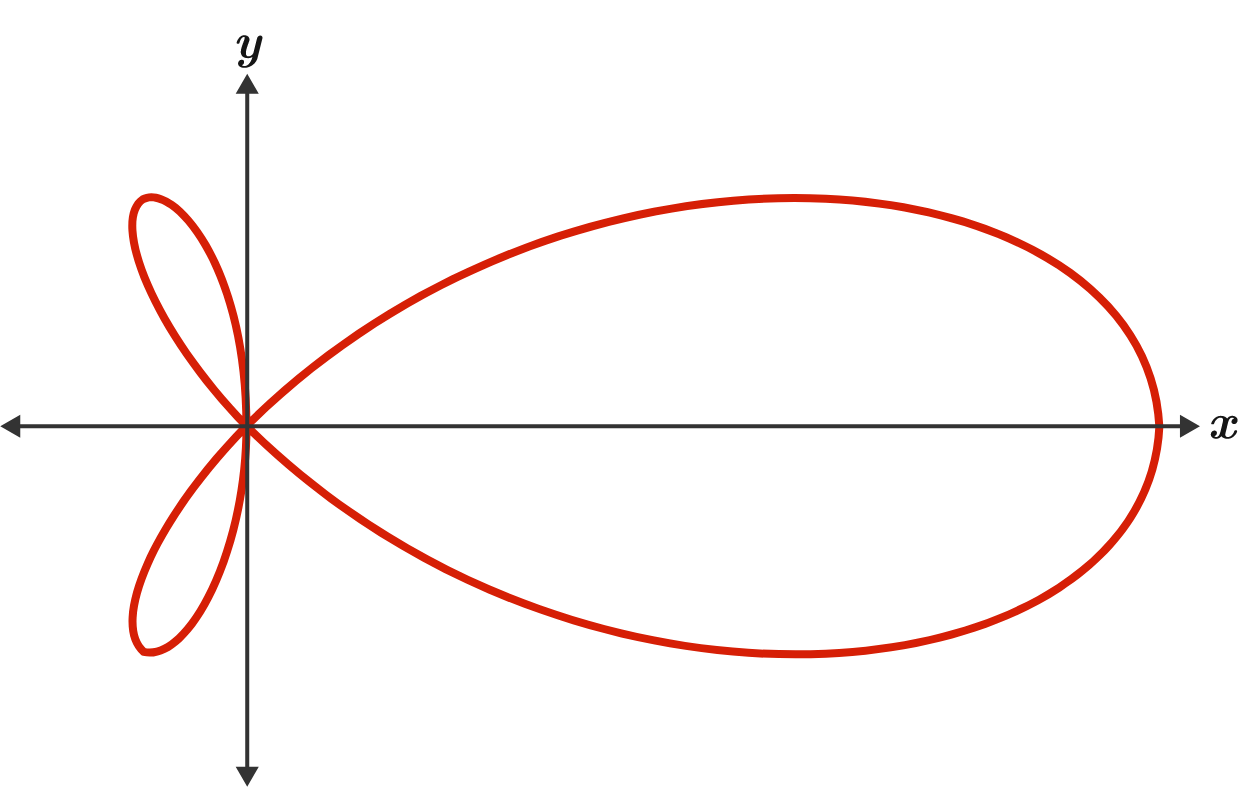# Fishy Volume

Calculus Level 3

This is the graph of $r=\frac{\sin4\theta}{\sin\theta}$ in polar coordinates.

Rotating the graph about the $x$-axis produces a volume of $\frac{a}{b}\pi$, where $a$ and $b$ are coprime positive integers.

What is $a+b?$×